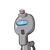# Write all the letters of the Word ‘TEXTBOOK’On each casd & firm the card upside down if youPick up a card from it, find the pr

Write all the letters of the Word ‘TEXTBOOK’On each casd & firm the card upside down if youPick up a card from it, find the probability that It is the letter ‘T’​

### 1 thought on “Write all the letters of the Word ‘TEXTBOOK’On each casd & firm the card upside down if youPick up a card from it, find the pr”

1.$$\frac{1}{4}$$

Explanation:

Total words: 8

Total T: 2

Probability=

$$\frac{no \: of \: successful \: outcomes}{total \: no \: of \: outcomes}$$

Therefore,

P(t) =

$$\frac{2}{8}$$

=

$$\frac{1}{4}$$